top of page
Search

# FEA Brain Teasers - FEA Exam And Interview Prep - Mechanics

Updated: Nov 7, 2022

Welcome to the second post in our three-part series featuring all our Brain Teasers that we posted weekly on our LinkedIn for two years! This week’s collection of Brain Teasers focuses on mechanics-related questions, further sorted by subject. The answers to these questions as well as the explanations are shown after all the questions. These Brain Teasers make for excellent practice for technical interviews or for anyone looking to reinforce their FEA knowledge. Let us know how many you get right! Also, stay tuned next week for Part 3!

Part 1: Materials

Part 3: Analysis

## Questions

### Stress:

1.2.3.4.5.6.7.8.9.10.### Displacement:

11.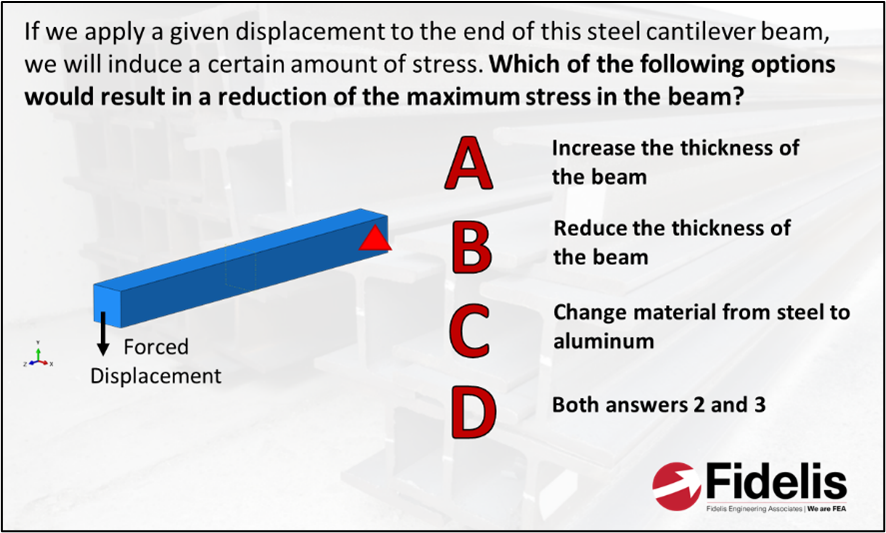12.### Forces and Moments:

13.14.### Failure:

15.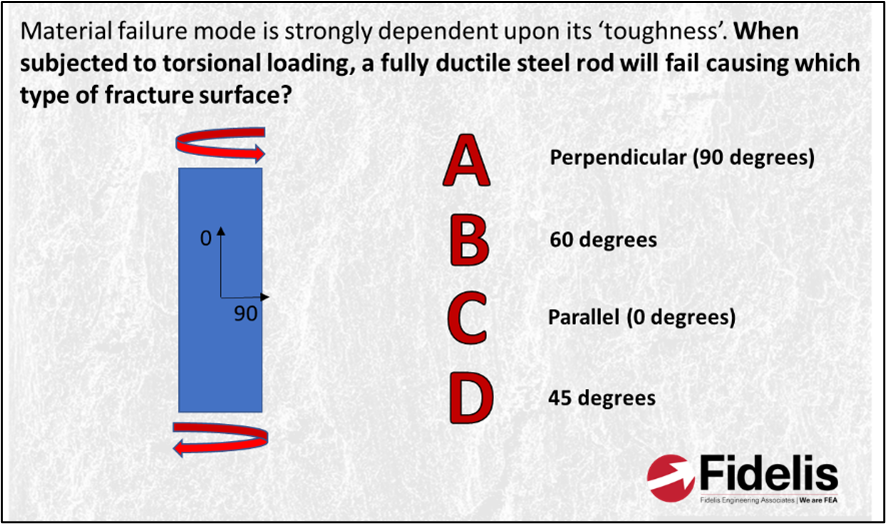16.### Pressure:

17.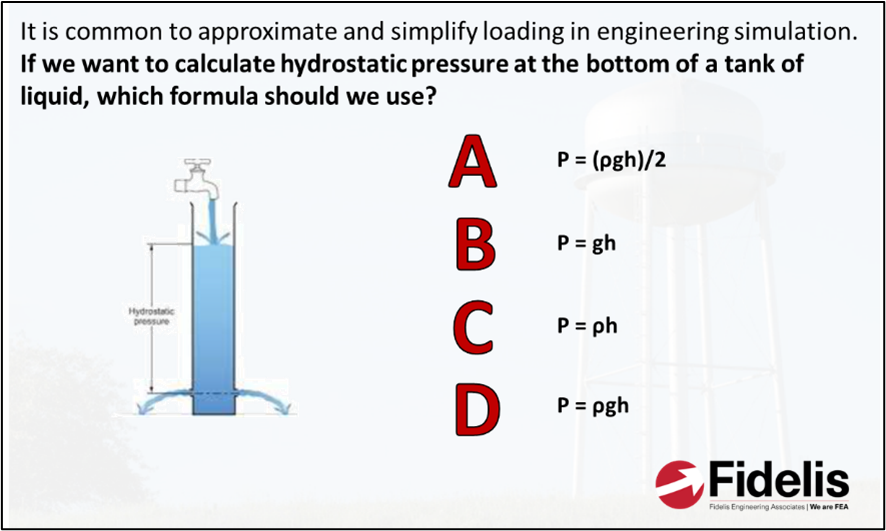18.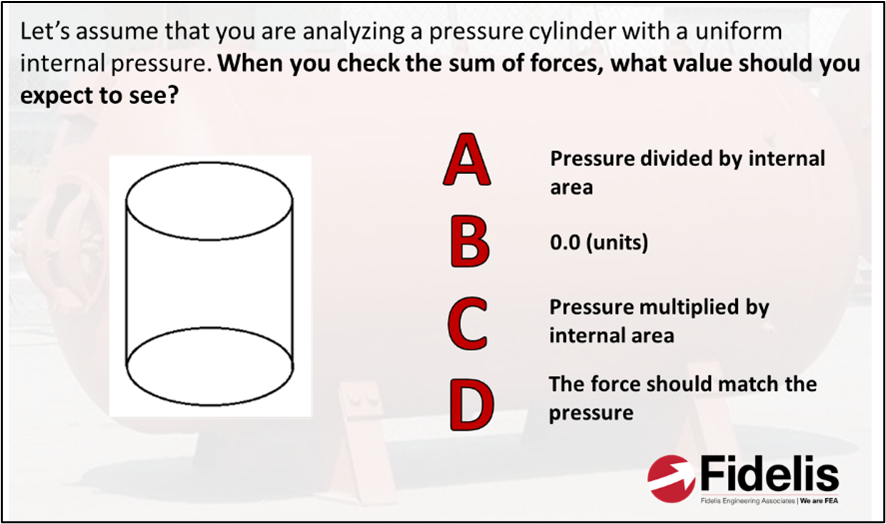19.### Dynamics:

20.21.### Nonlinear Geometry:

22.23.### Fatigue:

24.25.26.### Other:

27.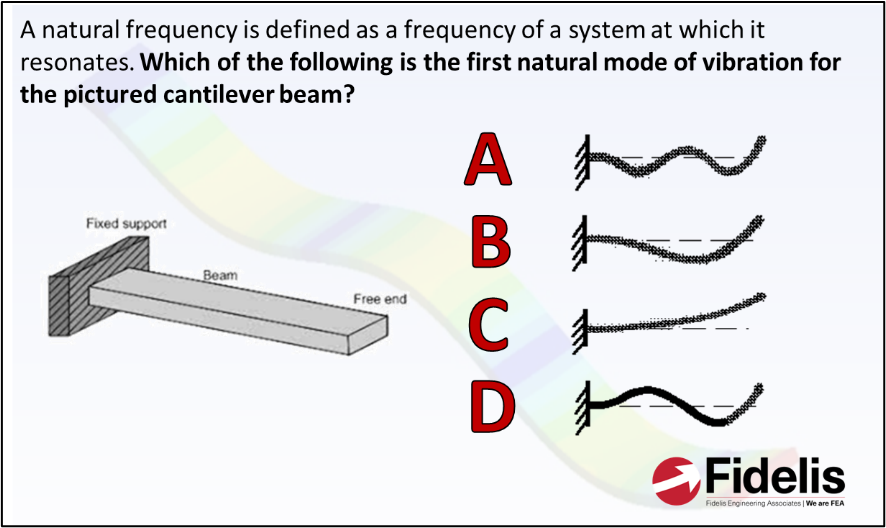28.29.1.2.Von Mises stress is a scalar representation of the deviatoric stress state in the specimen or component. It can be ‘signed’ in some FEA post-processors, but that’s really just a way to visualize whether tensile or compressive stresses dominate.

3.4.Residual stress is defined as stresses that remain even without external load. Some common applications where you could find residual stresses are welded components and cold-worked materials. A cold-worked material that has high residual stresses usually has higher hardness but is also more brittle.

5.As you go down the bar, less weight needs to be supported, so the stress in the hanging bar decreases linearly from top to bottom when suspended under gravity.

6.Assuming the modulus of both steels is identical, the stresses would be unaffected by the change of steel in a linear-elastic analysis. Of course, the stiffness of different steels can vary by a few percent either way, but that was not the spirit in which the question was posed.

7.Barring any nonlinearity, the stresses in the beams are the same. The relative stiffness of them means that steel deflects around 1/3 of the amount that the aluminum does.

8.Maximum shear stress on the surface is both parallel and perpendicular to the axis of the shaft, while maximum principal stress is at 45 degrees. That’s why we see ductile shafts typically breaking with a flat fracture while brittle shafts show more of a spiral shape.

9.This was a bit of a tough question since you had to calculate it to be sure. Although the hollow shaft uses significantly less material (just over half), it has a much larger polar moment of inertia than the solid shaft, hence why we often use quite thin-walled tubes for prop shafts in large vehicles.

Shear stress = Torque * radius / polar moment of inertia

Solid shaft:

J = (pi*D4)/32 = 15,700 mm4

Shear stress = 636.94 MPa

Hollow shaft:

J = (pi*(D4-d4))/32 = 22,630 mm4

Shear stress = 552.36 MPa

10.Note that double arrows signify moment, not a force! The I-beam ends up being the strongest due to it having the most material away from the neutral axis and therefore a higher second moment of area. The cross-sections were actually already ordered from highest to lowest second moment of area.

11.Since we’re applying a displacement instead of a load, stresses can be reduced by making the beam less stiff. Both reducing the thickness and changing the material to aluminum reduces the stiffness in the beam, reducing the maximum stress.

12.The formula for angle of twist is theta = TL/JG. Increasing the length and decreasing the moment of inertia would increase twist angle. Since steel has a higher shear modulus, twist angle would decrease. While it is possible that the twist angle can be reduced using a combination of all three if the increased length and change in moment of inertia has less of an effect than changing the material, only changing the material to steel would always reduce the twist angle.

13.Because the moment is in the middle, both sides must react the same force magnitude to maintain static equilibrium. However, the fixed end would also see a moment.

14.Per Table 8.1 in Roark's Formulas for Stress and Strain, the formula for the moment at fixed end "A" is -Fa(L-a)^2/L^2. If we were to take the absolute value and set F and L equal to 1, the magnitude of the moment simplifies to a(1-a)^2. If we expand and take the derivative of this, we get (3a-1)(a-1). This gives us the two solutions a=1/3 and a=1 as local minimums/maximums. Out of these, only a=1/3 is a local maximum since the second derivative (6a-4) is negative at this point. Substitute L back in and the answer is a=L/3. Try modeling this and see for yourself! a = L/2 would be correct if we were looking for the magnitude of the moment at the point of the applied load.

15.The fracture surface is a function of the failure mode and, in this case, we have ductile failure that is driven by shear stresses. The plane of maximum shear is perpendicular to the rod, hence the fracture surface is flat. A brittle fracture would occur at 45 degrees – the direction of maximum principal stress!

16.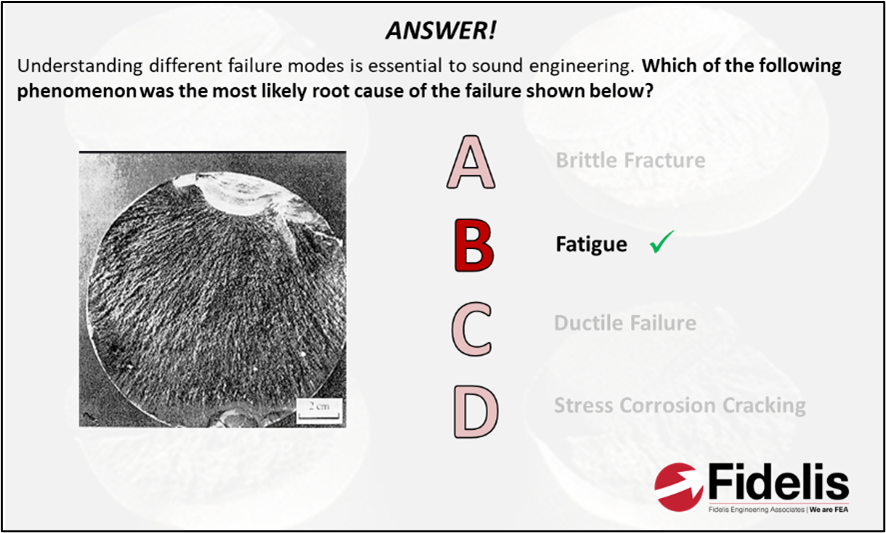Since we said ‘root cause’, we were looking for fatigue, but the final failure was the result of brittle fracture.

17.Rho being fluid density, g being gravitational acceleration and h being the height of the column.

18.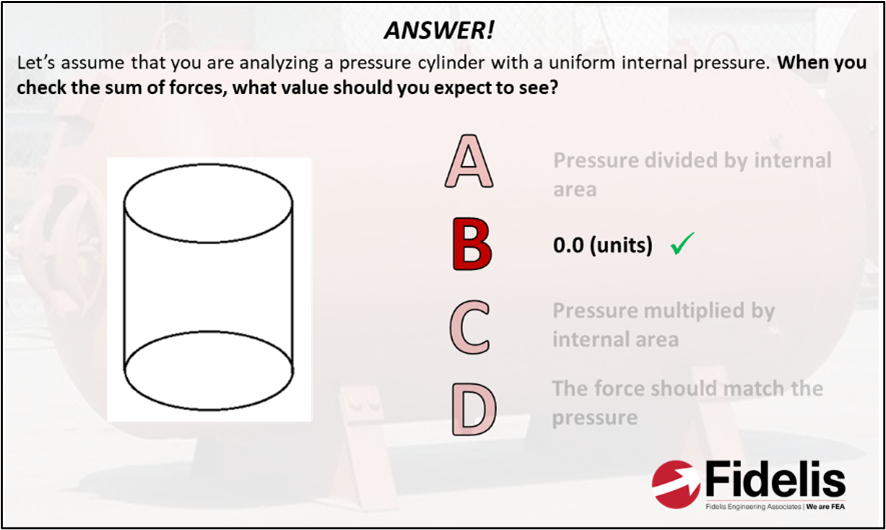In a static model, the sum of reaction forces should be equal and opposite to the applied load. In this case, the reaction forces in the pressure cylinder are equal and opposite to the applied internal pressure. When these are added up, the sum of forces should be 0.

19.As shown in the two formulas above, hoop stress is double the longitudinal stress in an idealized thin-walled pipe.

20.While damping and air resistance could potentially play an effect, since we’re in explicit and there was no mention of that in the setup, we have both objects hitting the ground at the same time. Interestingly, we needed double precision turned on to avoid rounding errors in our test case, so keep that in mind with all of your explicit analyses.

21.When a wheel is spinning at constant angular velocity, the acceleration is directly toward the center of the rim.

22.Without the effect of stress stiffening, which requires progressive recalculation of the stiffness matrix, the only resistance to deformation we have is bending stiffness. And in a thin wire, that is very low. Check out the example above. This is a short wire, fixed at both ends, with an arbitrary force applied in the center. The case without consideration of nonlinear geometry predicts ~6.5x deflection.

23.Nonlinear geometry captures the effects of shape change in the stiffness matrix. If we accounted for geometric nonlinearity, Beam 2 would have a significant amount of tension due to the shape change, which would decrease the deflection in the beam. There would be no tension in Beam 1 since it is allowed to translate horizontally. However, since nonlinear geometry is turned off in this case, the tension component would not exist in Beam 2, meaning both beams deform the same.

24.We're claiming that Initiation-Propagation-Rupture is the 'correct' answer, based on the commonly accepted Paris Law model. However, there could be argument that nucleation is an additional phase that comes between initiation and propagation. Great talking point for those interested.

25.As mean stress becomes more positive, the fatigue life curve shifts down, which has a negative effect on the fatigue life of a component (when compared to one exposed to equivalent, fully reversing stress amplitude).

26.For a given stress level, high stress gradient actually contributes to enhanced fatigue life. Stress gradient at a notch has a significant impact on the volume of material that is ‘stressed’ as well as the driving force for cracks that have initiated. Many commercial fatigue solvers account for the stress gradient or notch effect, for example fe-safe utilizes the Theory of Critical Distance while FEMFAT uses guidelines laid out in FKM.

27.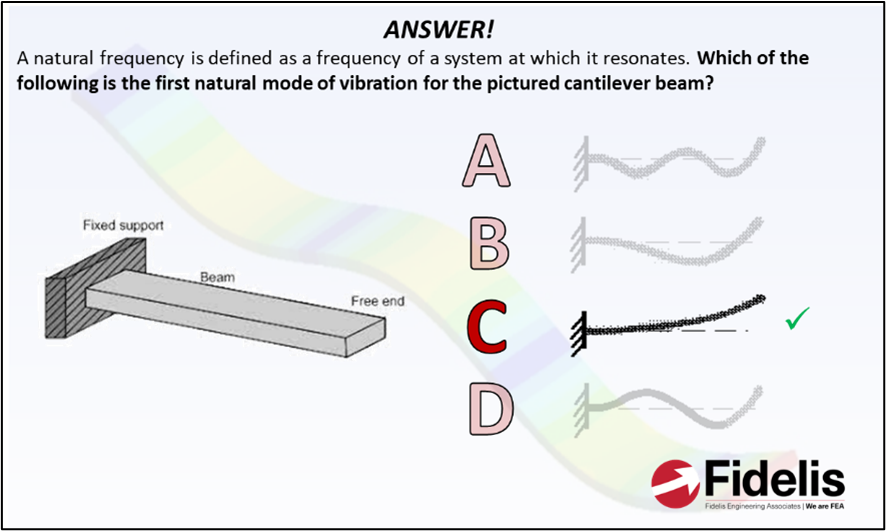The first natural mode of vibration occurs at the lowest frequency and has the simplest shape.

28.The heat flow rate is directly proportional to conductivity, area, and temperature difference and inversely proportional to length. Since aluminum has a higher conductivity than steel, the heat flow rate will increase by changing the material to aluminum. All the other answers will decrease the heat flow rate.

29.The Reynolds number defines the ratio between inertial and viscous forces in a fluid, while the rest are relevant to diffusivity, buoyancy, and heat transfer respectively.

For more awesome FEA related fun and content, check out our LinkedIn page!

1,181 views
bottom of page